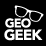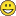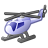# Convert polygon outlines into points

8263
13
12-26-2014 04:53 PMOccasional Contributor

Hi,

Is there anyway to convert polygon outlines into points, preferably with the ability to decide the gap of each point to be placed (just like the "construct points" from lines tool)?

The purpose is for creating service areas around polygons in Network Analyst. Or if there is another method of doing this besides the conversion do let me know!

I am using ArcGIS 10.2.2.

Thanks!

Tags (2)
1 Solution

Accepted SolutionsbyEsri Esteemed Contributor

To create points on the outline of a polygon with a defined interval, you could use some Python code:

```import arcpy

fc_in = r"C:\Forum\test.gdb\Polygon"
fc_out = r"C:\Forum\test.gdb\Points"
interval = 50

pnts = []
with arcpy.da.SearchCursor(fc_in, ("SHAPE@")) as curs:
for row in curs:
polygon = row
outline = polygon.boundary()
d = 0
while d < outline.length:
pnt = outline.positionAlongLine(d, False)
pnts.append(pnt)
d += interval

arcpy.CopyFeatures_management(pnts, fc_out)```

Change the path to input (polygon) and output (points) featureclass on line 3 and 4. Specify the interval of locating points on the outline on line 5 and run the code...

13 RepliesbyEsri Esteemed Contributor

To create points on the outline of a polygon with a defined interval, you could use some Python code:

```import arcpy

fc_in = r"C:\Forum\test.gdb\Polygon"
fc_out = r"C:\Forum\test.gdb\Points"
interval = 50

pnts = []
with arcpy.da.SearchCursor(fc_in, ("SHAPE@")) as curs:
for row in curs:
polygon = row
outline = polygon.boundary()
d = 0
while d < outline.length:
pnt = outline.positionAlongLine(d, False)
pnts.append(pnt)
d += interval

arcpy.CopyFeatures_management(pnts, fc_out)```

Change the path to input (polygon) and output (points) featureclass on line 3 and 4. Specify the interval of locating points on the outline on line 5 and run the code...Occasional Contributor

Thanks!

It works just fine!Appreciate the help.New Contributor II

Hi,

Thank you for this script. It works perfectly.

Is there a way i can add an attribute value from the original line to the associated points that are created? I would like to be able to see which points are on each line in a multiple feature featureclass.

Many thanks for any suggestions.

JamesbyEsri Esteemed Contributor

I think it would be something like this (did not test it though):

```import arcpy
import os

fc_in = r"C:\Forum\test.gdb\Polygon"
fc_out = r"C:\Forum\test.gdb\Points"
interval = 50
fld_in = "TheNameOfTheFieldYourWantToIncludeInYourOutput"

# determine the input spatial reference
sr = arcpy.Describe(fc_in).spatialReference

# create the empty output featureclass
fc_ws, fc_name = os.path.split(fc_out)
arcpy.CreateFeatureclass_management(fc_ws, fc_name, "POINT", spatial_reference=sr)

# add the field to the output
fld = arcpy.ListFields(fc_in, wild_card=fld_in)
arcpy.AddField_management(fc_out, fld_in, fld.type, fld.precision, fld.scale, fld.length)

# start output cursor
with arcpy.da.InsertCursor(fc_out, ("SHAPE@", fld_in)) as curs_out:
with arcpy.da.SearchCursor(fc_in, ("SHAPE@", fld_in)) as curs_in:
for row_in in curs_in:
polygon = row_in
outline = polygon.boundary()
value = row_in
d = 0
while d < outline.length:
pnt = outline.positionAlongLine(d, False)
curs_out.insertRow((pnt, value, ))
d += interval```Occasional Contributor II

helo, i get en error:

with arcpy.da.SearchCursor(fc_in, ("SHAPE@")) as curs:

AttributeError: 'module' object has no attribute 'da'

what wrong?Occasional Contributor

Hi YYC,

I read that the da module only works in version 10.1 onwards. Could it be that you are using a lower version?

SeeOccasional Contributor II

hi

i work on arcmap 10.3.

since i run your script (didnt worked out) i gen en error message and no other script can run at all:

Traceback (most recent call last😞

File "C:\yaron\shonot\software\gis\tools\YARON_SCRIPTS\ExportToJPG.py", line 2, in <module>

import arcpy,os,sys,string

File "C:\Program Files (x86)\ArcGIS\Desktop10.3\ArcPy\arcpy\__init__.py", line 24, in <module>

from arcpy.toolbox import *

File "C:\Program Files (x86)\ArcGIS\Desktop10.3\ArcPy\arcpy\toolbox.py", line 15, in <module>

from .ddd import ASCII3DToFeatureClass as ASCII3DToFeatureClass_3d

File "C:\Program Files (x86)\ArcGIS\Desktop10.3\ArcPy\arcpy\ddd.py", line 23, in <module>

from arcpy.arcobjects.arcobjectconversion import convertArcObjectToPythonObject

File "C:\Program Files (x86)\ArcGIS\Desktop10.3\ArcPy\arcpy\arcobjects\__init__.py", line 19, in <module>

arcobjectconversion.initialize_conversion_mapping()

File "C:\Program Files (x86)\ArcGIS\Desktop10.3\ArcPy\arcpy\arcobjects\arcobjectconversion.py", line 26, in initialize_conversion_mapping

from .._mapping import MapDocument, PageLayout, DataFrame, DataFrameTime, DataDrivenPages, \

File "C:\Program Files (x86)\ArcGIS\Desktop10.3\ArcPy\arcpy\_mapping.py", line 8, in <module>

with arcpy.da.SearchCursor(fc_in, ("SHAPE@")) as curs:

AttributeError: 'module' object has no attribute 'da'byEsri Esteemed Contributor

I don't think the script I posted can have this effect on your behavior of arcpy modules in ArcGIS 10.3. Are you sure nothing else happened at your system? Did you perhaps install ArcGIS Pro and python 3.4? There might be something wrong with the definition of your paths.New Contributor III

Hi,

I need to solve a similar problem, but not only for polygon features but also for line features.

Is there any way to implement this useful script in ArcGIS 10.0, which lacks the arcpy.da module (Data Access Module)?